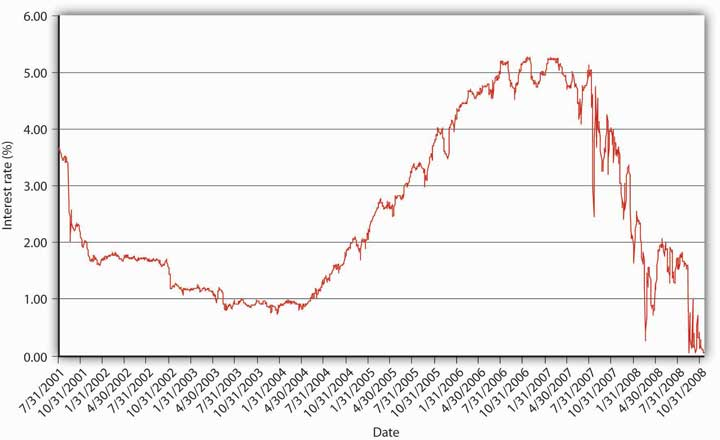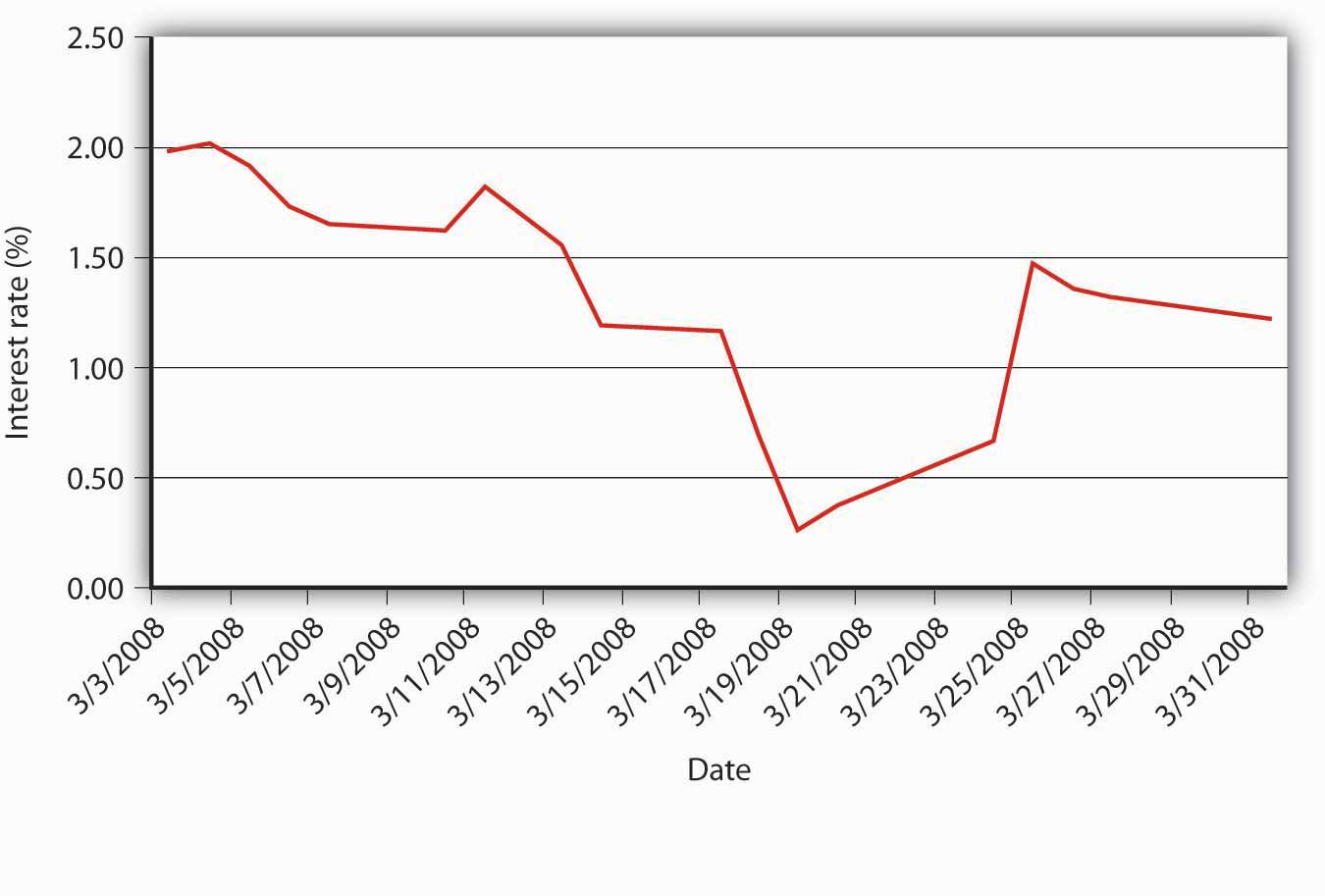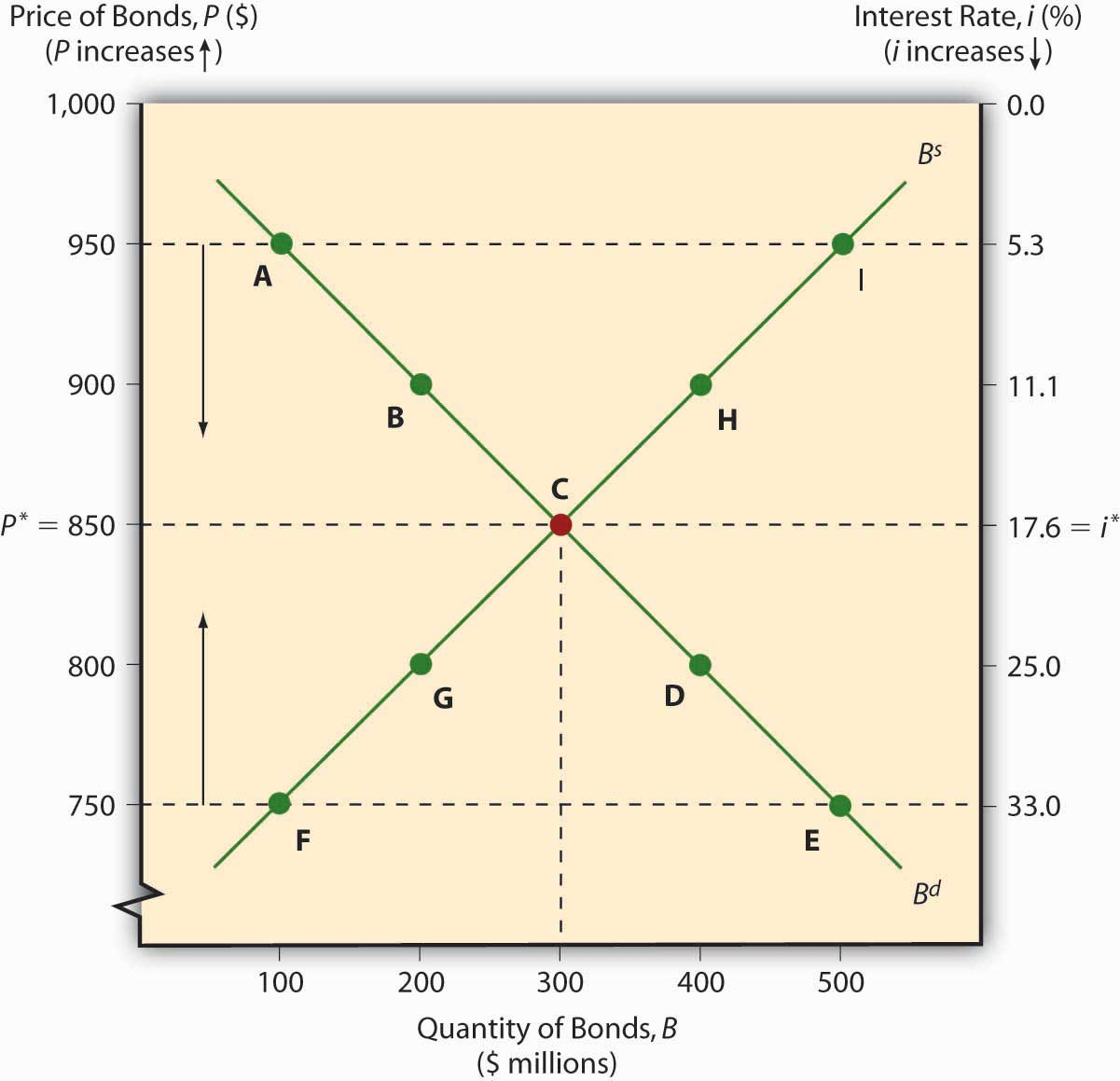This is “Interest Rate Fluctuations”, section 5.1 from the book Finance, Banking, and Money (v. 1.1). For details on it (including licensing), click here.

Has this book helped you? Consider passing it on:
Creative Commons supports free culture from music to education. Their licenses helped make this book available to you.
DonorsChoose.org helps people like you help teachers fund their classroom projects, from art supplies to books to calculators.

## 5.1 Interest Rate Fluctuations

### Learning Objective

1. As a first approximation, what causes the interest rate to change?

If you followed the gist of Chapter 4 "Interest Rates", you learned (we hope!) about the time value of money, including how to calculate future value (FV), present value (PV), yield to maturity, current yield (the yield to maturity of a perpetuity), rate of return, and real interest rates. You also learned that a change in the interest rate has a profound effect on the value of assets, especially bonds and other types of loans, but also equities and derivatives. (In this chapter, we’ll use the generic term bonds throughout.) That might not be a very important insight if interest rates were stable for long periods. The fact is, however, interest rates change monthly, weekly, daily, and even, in some markets, by the nanosecond. Consider Figure 5.1 "Yields on one-month U.S. Treasury bills, 2001–2008" and Figure 5.2 "Yields on one-month U.S. Treasury bills, March 2008". The first figure shows yields on one-month U.S. Treasury bills from 2001 to 2008, the second shows a zoomed-in view on just March 2008. Clearly, there are long-term secular trends as well as short-term ups and downs.

Figure 5.1 Yields on one-month U.S. Treasury bills, 2001–2008Figure 5.2 Yields on one-month U.S. Treasury bills, March 2008You should now be primed to ask, Why does the interest rate fluctuate? In other words, What causes interest rate movements like those shown above? In this aptly named chapter, we will examine the economic factors that determine the nominal interest rate. We will ignore, until the next chapter, the fact that interest rates differ on different types of securities. As we’ll learn in Chapter 6 "The Economics of Interest-Rate Spreads and Yield Curves", interest rates tend to track each other, so by focusing on what makes one interest rate move, we have a leg up on making sense of movements in the literally thousands of interest rates out there in the real world. Another way to think about this is that, in this chapter, we will concern ourselves only with the general level of interest rates, which economists call “the” interest rate.

The keys to understanding why “the” interest rate changes over time are simple price theory (supply and demand), the theory of asset demand, and the liquidity preference framework of renowned early twentieth-century British economist John Maynard Keynes.http://www-history.mcs.st-andrews.ac.uk/Biographies/Keynes.html Like other types of goods, bonds and other financial instruments trade in markets. The demand curve for bonds, as for most goods, slopes downward; the supply curve slopes upward in the usual fashion. There is little mystery here. The supply curve slopes upward because, as the price of bonds increases (which is to say, as we learned in Chapter 4 "Interest Rates", as their yield to maturity decreases), ceteris paribus, borrowers (sellers of securities) will supply a higher quantity, just as producers facing higher prices for their wares will supply more cheese or automobiles. As the price of bonds falls, or as the yield to maturity that sellers and borrowers offer increases, sellers and borrowers will supply fewer bonds. (Why sell ’em if they aren’t going to fetch much?) The demand curve for bonds slopes downward for similar reasons. When bond prices are high (yields to maturity are low), few will be demanded. As their price falls (their yields increase), investors (buyers) want more of them because they are increasingly good deals.

The market price of a bond and the quantity that will be traded is determined, of course, by the intersection of the supply and demand curves, as in Figure 5.3 "Equilibrium in the bond market". The equilibrium price prevails in the market because, if the market price were temporarily greater than p*, the market would be glutted with bonds. In other words, the quantity of bonds supplied would exceed the quantity demanded, so sellers of bonds would lower their asking price until equilibrium was restored. If the market price temporarily dipped below p*, excess demand would prevail (the quantity demanded would exceed the quantity supplied), and investors would bid up the price of the bonds to the equilibrium point.

Figure 5.3 Equilibrium in the bond marketAs with other goods, the supply and demand curves for bonds can shift right or left, with results familiar to principles (“Econ 101”) students. If the supply of bonds increases (the supply curve shifts right), the market price will decrease (the interest rate will increase) and the quantity of bonds traded will increase. If the supply of bonds decreases (the supply curve shifts left), bond prices increase (the interest rate falls) and the equilibrium quantity decreases. If the demand for bonds falls (the demand curve shifts left), prices and quantities decrease (and the interest rate increases). If demand increases (the demand curve shifts right), prices and quantities rise (and the interest rate falls).

### Key Takeaways

• The interest rate changes due to changes in supply and demand for bonds.
• Or, to be more precise, any changes in the slopes or locations of the supply and/or demand curves for bonds (and other financial instruments) lead to changes in the equilibrium point (p* and q*) where the supply and demand curves intersect, which is to say, where the quantity demanded equals the quantity supplied.